Current Commentary Coming Next
 TV Series Theory LA in the 1970s: Experimental Finance Part I: The Long Goodbye Comes of Age March 11, 2013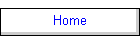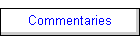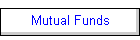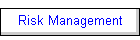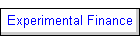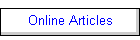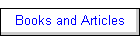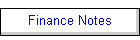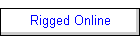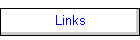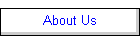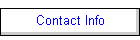This is the HTML output of a Mathematica notebook that appeared in  Economic and Financial Modeling with Mathematica, Hal R. Varian, ed., TELOS/Springer-Verlag, 1993. There are several formatting issues with the HTML and it is suggested that readers with Mathematica or the Mathematica reader view the Mathematica notebook version of this paper.

Option Valuation

by

Ross M. Miller
Niskayuna, NY 12309

### Introduction

Although financial computation is often viewed as an exercise in number crunching, the emergence of a new breed of financial "rocket scientists" has expanded the role of computers in finance to include not only numerical manipulations, but also structural manipulations. Investment houses now routinely "slice and dice" securities such as mortgages, government bonds, and even the infamous "junk bonds," to engineer their cash flows to meet particular risk/return criteria. While spreadsheet programs and traditional programming languages (e.g., FORTRAN and C) continue to play an important role in financial computation, symbolic programming languages, i.e., languages that manipulate both the numbers and the symbols with which financial structures are represented, are taking hold as a way of dealing with the increasing complexity of the financial world. Indeed, some of the more innovative investment houses around the world have been using LISP and Smalltalk since the mid-1980's to handle a variety of difficult valuation and design problems.

This chapter contains a redesigned version of a suite of option valuation tools that the author originally developed in LISP as part of a comprehensive textbook on the application of object-oriented and artificial intelligence technology to finance analysis (Miller, 1990a), and some of which were included in the premiere issue of the Mathematic Journal (Miller, 1990b). It is a testament to Mathematica's flexibility that even the most complex LISP-based tool developed in conjunction with that textbook ports easily to Mathematica. This chapter contains a brief introduction to option valuation; however, a more complete introduction to the topic can be found in any of several sources. The original exposition of the Black-Scholes model appears in Black and Scholes, 1973 and an excellent adaptation of the Cox-Ross-Rubinstein binomial model appears in Cox and Rubinstein, 1985. An excellent textbook that covers a wide range of topics in option valuation is Hull, 1990.

This chapter takes a very general approach to the problem of financial valuation, utilizing object-oriented design methods to the extent that they are possible within Mathematica to achieve this generality. The traditional approach that economists have taken to computing has been to create completely separate programs or procedures for each model. In the object-oriented approach to computing the goal is to create general valuation procedures, called methods, that can operate on many different types of objects, in this case options. The advantage to the object-oriented approach is that the development of new financial instruments does not require programming new, ad hoc valuation procedures. Instead, existing objects that represent related financial instruments are updated to reflect any innovations in the newly-created instrument. Furthermore, once an object has been formally defined, other methods can be created to perform other functions, such as accounting, without starting from scratch.

The Mathematica functions developed in this chapter were designed with their pedagogical utility foremost in mind, especially when used interactively as a Mathematica notebook. Although we have tried to make them as computationally efficient as possible, in some instances speed has been sacrificed in favor of simplicity or elegance. In particular, the binomial model has been developed within a very general framework that is readily extensible to more complex valuation problems, but is in no way optimized for the binomial model. In addition, the tendency in this chapter is to use Mathematica's built-in algorithms even in cases where user-defined alternatives would be far more efficient. Finally, the functions developed in this chapter have been designed for interpreted rather than compiled use.

### The Black-Scholes Model

The Black-Scholes model provides a direct way of valuing a call option for common stock. A call option is an option to buy stock at a pre-specified exercise or strike price prior to a given expiration date. (An option which can only be exercised on its expiration date is known as a European option, while one that can be exercised at any time prior to expiration is known as an American option. Most exchange-traded stock options in the U.S. are American options. Except in special cases, the Black-Scholes model must be modified to deal with the possibility of early exercise.)

If the market price of the stock is greater than the exercise price when the expiration date arrives, then the value of the option will be equal to the payoff that can be created by buying the stock at the exercise price and then immediately selling the stock at its market price. Otherwise, it will not pay to exercise the option, and so it will expire with zero value. The payoff pattern of an option is easily modeled in Mathematica by the function CallPayoff as follows:

`CallPayoff[price_,strike_] = Max[0,price-strike]`
`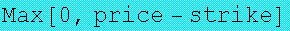`

For example, consider the payoff function for a call option that gives the holder the option to buy a share of DEC stock at a price of \$60 on the third Friday in June. If the price of DEC stock is \$80 on expiration in June, then the option will pay off \$20; however, if DEC stock is below \$60, the option will be worth \$0 as it will not pay to exercise it. This payoff function is readily plotted as follows:

`Plot[CallPayoff[x,60],{x,0,120}]`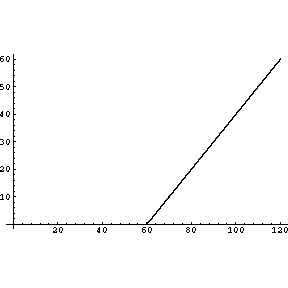`     -Graphics-`

Similiarly, the payoff function for a put option, i.e., an option to sell a share of stock at a predetermined exercise price (in this case, \$60) can be defined and plotted as follows:

`PutPayoff[price_,strike_] = Max[0,strike-price]`
`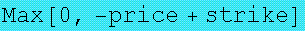`

`Plot[PutPayoff[x,60],{x,0,120}]`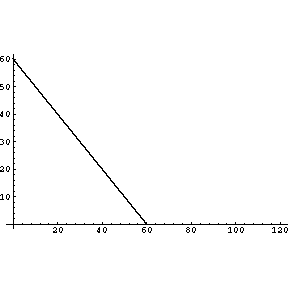`     -Graphics-`

Hence, at a price below the exercise price, it will pay to purchase the stock and then use the put option to sell it at the exercise price; otherwise, the put option is worthless.

A payoff diagram, such as those shown above, only provides the value of an option at a boundary--the moment the option expires at the close of trading on the expiration date. Determining the value of the option prior to expiration can be reduced to taking the current price of the stock, which is presumably known, and projecting it forward in time to the expiration date. Because of the many factors that can affect the price of a stock, this projection must be made probabilistically rather than deterministically. In the Black-Scholes model, it is assumed that the percentage change in the stock price (its rate of return when no dividends are paid) follows a Weiner process (i.e., a random walk) with a known drift and standard deviation, so that on the expiration date, the stock price has a lognormal distribution with a known mean and variance.

Although the lognormality assumption of the Black-Scholes model cannot be expected to hold in any but the most contrived situations, the model (and its variants) can be used to predict option prices with great accuracy under a broad range of real-world situations. Indeed, most traders on the floors of options exchanges carry computerized "crib sheets" with them that give the theoretical value of the options in which they trade so that they may identify options whose prices are temporarily mispriced relative to the model.

Under the further assumptions that the stock pays no dividends and will only be exercised, if at all, upon expiration, the Black-Scholes model yields a closed-form equation for the value of the call option that is easily modelled in Mathematica. Although this derivation was a notable technical feat when it was first derived around 1970, it is now a standard application of stochastic calculus. (The use of Mathematica to do stochastic calculus appears elsewhere in this book.)

Because it is relatively complex, the Black-Scholes formula is best expressed in terms of an auxiliary function. Both the Black-Scholes formula and the auxiliary functions take five arguments as follows:

p = current price of the stock
k = exercise price of the option
sd = volatility of the stock (standard deviation of annual rate of return)
r = continuously compounded risk-free rate of return, e.g., the return on
U.S. Treasury bills with very short maturities
t = time (in years) until the expiration date

The critical feature of the Black-Scholes and related option valuation models is that the value of the option depends only on the standard deviation of the stock's rate of return and not upon its expected value. That is because the model is based on an arbitrage argument in which any risk premium above the risk-free rate of return is cancelled out.

Using the five variables given above, we can define the auxiliary function, AuxBS, and the Black-Scholes valuation function, BlackScholes, as follows:

```
AuxBS[p_,k_,sd_,r_,t_] = (Log[p/k]+r t)/
(sd Sqrt[t])+
.5 sd Sqrt[t]```
`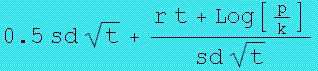`

```BlackScholes[p_,k_,sd_,r_,t_] =
p  Norm[AuxBS[p,k,sd,r,t]]-
k Exp[-r t] (Norm[AuxBS[p,k,sd,r,t]-sd Sqrt[t]])```
``

The function Norm is the cumulative normal distribution function and can be defined for numerical arguments as follows:

```
Norm[z_?NumberQ]:= N[0.5 + 0.5 Erf[z/Sqrt]]```

A separate definition of the derivative of Norm used for symbolic valuation will be given in the following section on risk management.

Notice that because BlackScholes is defined using the immediate assignment operator, =, it contains the full Black-Scholes formula with the actual values from AuxBS appropriately substituted as shown by the output given above.

We are now ready to compute the dollar value for stock options by calling the function BlackScholes with numerical arguments. For example, to continue the above dialog, we might wish to find the value of a call option on DEC stock with an exercise price of 60 assuming that the current price of DEC is 58 1/2, the time until expiration is 0.3 years, the volatility of DEC stock is 29%, and the continuously compounded risk-free rate of return is 4% as follows:

`BlackScholes[58.5,60.,0.29,0.04,0.3]`
`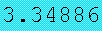`

Using the graphic capabilities of Mathematica one can easily explore the various qualititative properties of the Black-Scholes formula and even go so far as to animate them. Two parameters of the model that are certain to change as time passes are the stock price and, trivially, the time to expiration. The instantaneous dependence of the option value on the stock price for the DEC option can be shown as follows:

`Plot[BlackScholes[x,60.,0.29,0.04,0.3],{x,50,70}]`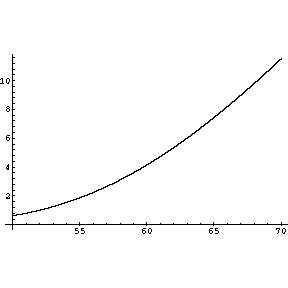`     -Graphics-`

Looking at the following 3D plot of option value as a function of both stock price and time until expiration, one can see how the curvature of the above graph diminishes as expiration approaches:

```Plot3D[BlackScholes[x,60.,0.29,0.04,y],{x,50.,70.},
{y,0.01,0.5},
ViewPoint->{1.450, -2.900, 0.810}]```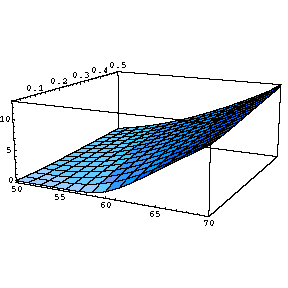`     -SurfaceGraphics-`

The sensitivity of the Black-Scholes model to the two parameters that characterize the "financial state of the world" can also be explored graphically using Mathematica. For example, although the Black-Scholes model assumes the volatility of the stock is constant, it will tend to fluctuate over the life of the option. The sensitivity of the value of an option to changes in volatility can be graphed as follows:

`Plot[BlackScholes[60.,60.,x,0.04,0.3],{x,0.2,0.4}]`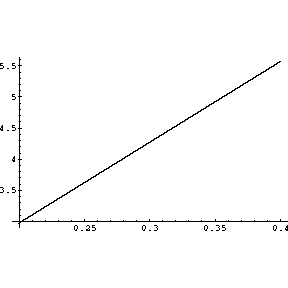`     -Graphics-`

This graph is not particularly exciting because over a broad range of volatilities the option value is nearly perfectly linear. Nonetheless, it is evidence of an important feature of the Black-Scholes model, i.e., that it provides an excellent approximation to the value of an option with variable volatility as long as the mathematical expectation of the volatility is known.

Although Mathematica's powerful and convenient graphics provide a qualitative insight into the Black-Scholes, the quantitative application of the model, especially to the area of risk management, is facilitated by applying the symbolic manipulation capabilities of Mathematica directly to the Black-Scholes formula. Such risk management applications are the focus of the next section.

### Risk Management using the Black-Scholes Model

The Black-Scholes model is useful not only because it provides a closed-form expression for the value of an option, but also because the sensitivity of the model to changes in its parameters, as represented by (partial) derivatives of the option valuation formula, can be expressed in closed form. The most important parameter that affects the value of an option is the price of the underlying stock. The partial derivative of option value with respect to stock price is known as delta .

Delta is a useful measurement of risk for an option because it indicates how much the price of the option will respond to a \$1 change in the price of the stock. For call options, delta can range from 0 to 1, i.e., the option may be insensitive to change in the stock price, may track it exactly, or may lie somewhere in between. Delta is a particularly useful gauge of the risk contained in a portfolio that contains more than one option on a given stock. In particular, the theoretical risk associated with the holding of a stock will be completely neutralized (in the very short run) if the overall dollar-weighted delta of a portfolio of options on that stock is equal to zero. This is because the portfolio delta gives change in portfolio value for a \$1 change in stock price, which is zero in this case. However, because delta is a partial derivative it assumes that all other variables are held constant and the change in stock price is relatively small, which will usually not be the case over even a short period of time. Additional measures of risk that either are derived later in this section or are contained in the accompanying Mathematica package can help the reader to get a handle on other changes that can influence option value.

Using the derivative function, D, that is built into Mathematica, it is easy to calculate symbolically any derivative of the BlackScholes function, including delta. Before these derivatives can be defined, however, it is first necessary to supply Mathematica with the derivative of the Norm function, since only the numerical value of Norm was provided above and its derivative frequently figures into derivatives of BlackScholes. In Mathematica, the derivative of Norm is defined as follows:

```
Norm'[z_] = N[(1/Sqrt[2 Pi])] Exp[-z^2/2]
```
`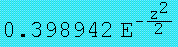`

The leading constant in the definition of this derivative is expressed as a number rather than being left in terms of Pi to ensure that full numerical conversion occurs when derivatives of the Black-Scholes formula are evaluated for a particular set of parameter values.

Then, the function that computes delta, Delta, becomes:

```
Delta[p_,k_,sd_,r_,t_] = D[BlackScholes[p,k,sd,r,t],p]
```
``

The assignment statement that defines Delta, like the one that defined BlackScholes, generates a formula for delta directly. It should be noted that the evaluation mechanism in Mathematica does not always generate formulas in their simplest form and, indeed, the expression output for Delta (and some other derivatives of BlackScholes) could be further simplified in the interests of computational efficiency as it is actually equal to Norm[AuxBS[p,k,sd,r,t].

When Delta is applied to the situation given above, we get the following:

```
Delta[58.5,60.,0.29,0.04,0.3]```
`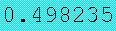`

Hence, for a \$1 increase in DEC stock, the price of this call option will increase by about 50 cents.

A plot of Delta against stock price provides a fundamental insight into option valuation as follows:

`Plot[Delta[x,60.,0.29,0.04,0.3],{x,20.,100.}]`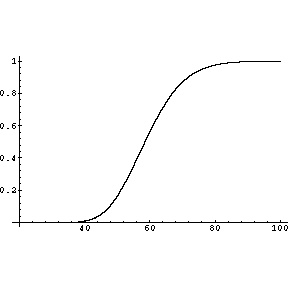`     -Graphics-`

For very low stock prices, i.e., when the call option is very much out of the money, delta is virtually zero indicating that the value of the option, which is also virtually zero, is insensitive to changes in the stock price because even a modest increase will have little effect of the likely outcome that the option will expire worthless. For very high stock prices, i.e., when the option is very much in the money, delta approaches one because the increase in the stock price dollar for dollar translates into an expected increase in the value of the option at expiration.

Other aspects of option value are as easy to investigate as delta using the symbolic math features of Mathematica. The sensitivity of the value of an option to time, theta, is derived as easily as delta was as follows:

```Theta[p_,k_,sd_,r_,t_] = -D[BlackScholes[p,k,sd,r,t],t]
```
`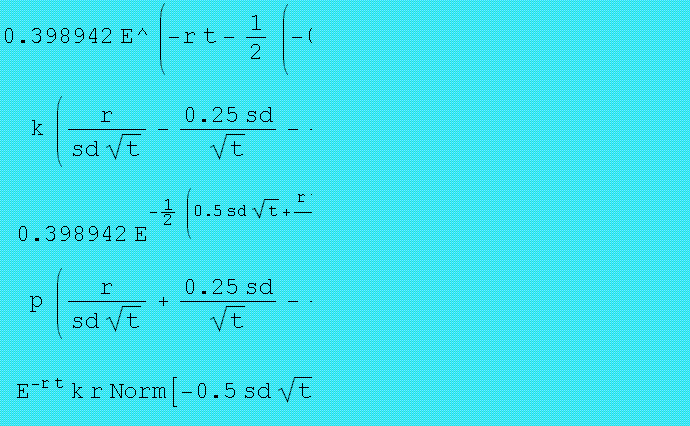`

`Plot[Theta[x,60.,0.29,0.04,0.3],{x,20.,100.}]`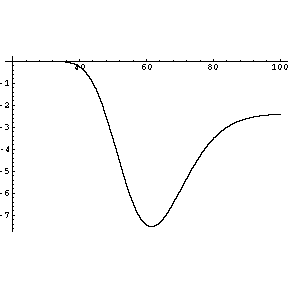`     -Graphics-`

By convention theta is taken to be the negative of the derivative with respect to time to expiration because moving forward in clock time decreases the time to expiration, hence theta gives the instantaneous change in the value of the option as time passes and all other parameters are unchanged. Because an option is more valuable the greater the time until expiration, theta is always negative. As the above plot clearly demonstrates, the time decay in the value of an option tends to be greatest when the stock price is close to the exercise price; however, as time passes this decay becomes more uniform across stock prices as the following 3D graph demonstrates:

```Plot3D[Theta[x,60.,0.29,0.04,y],{x,20.,100.},
{y,0.01,0.5},
ViewPoint->{0.520, -3.420, 1.810}]```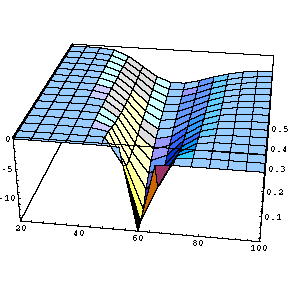`     -SurfaceGraphics-`

As was mentioned at the beginning of this section, the partial derivatives of the Black-Scholes formula can also be applied to an entire portfolio of options to determine the sensitivity of the value of the portfolio to changes in any or all of the variables that underlie the formula.

On a related note, in situations where an option's price is believed to be an accurate indication of its value, it can be desirable to "reverse engineer" the volatility of an option from its market price. The function ImpliedVolatility uses the built-in function FindRoot to solve numerically for the volatility of an option given its price as follows:

```
ImpliedVolatility[p_,k_,r_,t_,optionprice_] :=
sd /. FindRoot[BlackScholes[p,k,sd,r,t]==
optionprice,{sd,0.2}]
```

Hence, if we knew the price of the DEC option given above was 3.34886 we could verify that the volatility is 0.29 as follows:

`ImpliedVolatility[58.5,60.,0.04,0.3,3.34886]`
`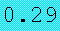`

Implied volatility is an extremely useful way of looking at options; indeed, some options on foreign currencies and other financial instruments are frequently quoted in terms of their implied volatility rather than by price, much as bonds are quoted by yield rather than price. There are many trading strategies that are designed to go either long or short volatility while limiting risk using the techniques described above.

As with any other group of related functions in Mathematica, the functions associated with the Black-Scholes model can be collected into a single Mathematica package that accompanies this book. The public part of this package, which appears before the Begin["`private`"] statement, provides access to these functions, as well as to a small database described later. This package includes not only the functions defined in this chapter but additional functions for popular measures of option value sensitivity--theta, kappa, rho, gamma, and (stock price) elasticity.

### Options as Objects

Mathematica provides now only numerical and symbolic manipulation capabilities for formulas, it also provides the basic tools needed to treat options and option-based securities as self-contained objects and to make the action of function depend on the type of object to which it is applied. Although the object manipulation capabilities of Mathematica fall short of those provided by object-oriented design toolkits, the core facilities for object creation and data abstraction are contained within Mathematica. This section will develop the tools for representing options as objects and the following section will exploit this representation to develop the technology for having a single Value function that is capable of evaluating a variety of options and other securities.

We will start by showing how the DEC call option (and its underlying DEC stock) can be represented as objects in Mathematica. The distinguishing features of a computational object are its properties. For example, the properties of the DEC option, DECFL, are that it is a call option on DEC stock, has an exercise price of \$60 and expires in June, which we have assumed to be 0.3 of a year away. In Mathematica, we can link these properties to the symbol DECFL as follows:

```Type[DECFL] ^= "call" ;
Asset[DECFL] ^= DEC ;
ExercisePrice[DECFL] ^= 60. ;
ExpirationTime[DECFL] ^= 0.3 ;```

The operator ^= is the known as UpSet and is used to make sure that Mathematica associates the value assignment with the "upvalue" DECFL rather than the head of the left-hand side of the assignments as it normally would. It is easy to check that these properties have been associated with DECFL as follows:

`?DECFL`
`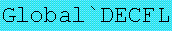`
`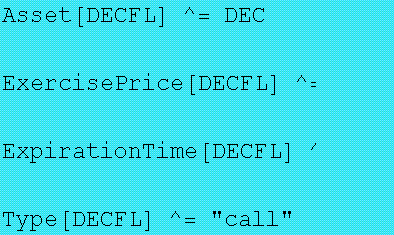`
```Global`DECFL

Asset[DECFL] ^= DEC

ExercisePrice[DECFL] ^= 60.

ExpirationTime[DECFL] ^= 0.3

Type[DECFL] ^= "call"```

As a convenience we will define an object constructor function, ConsObj, that takes a symbol and property list as its arguments:

```ConsObj[obj_,proplist_] :=
Do[Block[{propname=proplist[[2*i-1]],
propval=proplist[[2*i]]},
propname[obj]^=propval],
{i,Length[proplist]/2}]
```

The object, DECFL, can now be constructed as follows:

```ConsObj[DECFL,{Type,"call",
Asset,DEC,
ExercisePrice,60.,
ExpirationTime,0.3}]```

Notice that not all of the information needed to value the DECFL option is contained in its object description. In particular, the price and volatility of DEC stock are properties that must be "inherited" from the object representation of the stock. This object, DEC, can be constructed as follows:

```ConsObj[DEC,{Type,"stock",
Price,58.5,
Volatility,0.29}]
```

The properties of the DEC object are stored in Mathematica as follows:

`?DEC`
`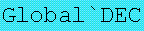`
`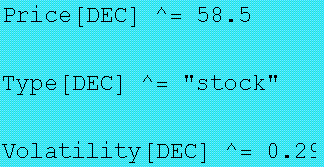`
```Global`DEC

Price[DEC] ^= 58.5

Type[DEC] ^= "stock"

Volatility[DEC] ^= 0.29```

Of course, these properties of the DECFL option that come from the underlying stock are not inherited automatically; Mathematica needs assignment rules to facilitate this inheritance as follows:

```AssetPrice[option_] := (AssetPrice[option] ^=
Price[Asset[option]]);
AssetVolatility[option_] := (AssetVolatility[option] ^=
Volatility[Asset[option]])```

This form for the assignment ensures that Mathematica associates the value with the option rather than the function. We can now test that these two new properties of the DECFL option have been properly inherited:

`AssetPrice[DECFL]`
`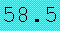`

`AssetVolatility[DECFL]`
``

Checking the values now associated with DECFL, we can see that these two new properties are listed:

`?DECFL`
``
`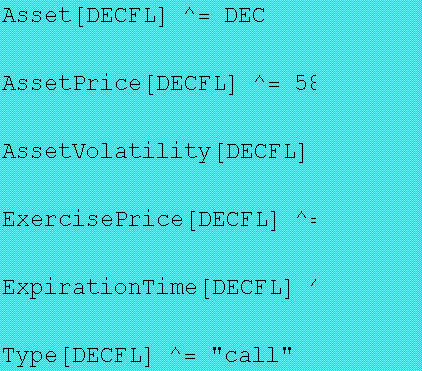`
```Global`DECFL

Asset[DECFL] ^= DEC

AssetPrice[DECFL] ^=
OptionValue`private`Price[OptionValue`private`Ass\
et[DECFL]]

AssetVolatility[DECFL] ^=
OptionValue`private`Volatility[OptionValue`private`Ass\
et[DECFL]]

ExercisePrice[DECFL] ^= 60.

ExpirationTime[DECFL] ^= 0.3

Type[DECFL] ^= "call"```

As noted above, Mathematica does not provide a full object-oriented design environment so these new "properties" are not generated automatically, but become part of the object description after their first use.
The final piece of information necessary to evaluate DECFL is the risk-free rate of return. Because this rate is assumed to be constant and can be applied to all options, it makes sense to define it as a global variable as follows:

`RiskFreeRate = 0.04`
`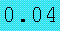`

It is now a simple matter to define a value function that takes an option's symbol as its argument and retrieves the necessary information to apply the Black-Scholes function defined above:

```Value[option_] := BlackScholes[AssetPrice[option],
ExercisePrice[option],
AssetVolatility[option],
RiskFreeRate,
ExpirationTime[option]]```
` `

We can now demonstrate that Value actually works when applied to DECFL:

`Value[DECFL]`
``

Of course, the Black-Scholes formula only applies to a limited number of options. In the next section we will look at other option valuation methods that can be applied to options in general, including put options, and see how the Value function can be appropriately extended.

### Valuing Options with Financial Decision Trees

When considering options for which the Black-Scholes model is not designed, e.g., American put options (options to sell that can be exercised early), there is usually no closed-form solution and closed-form approximations are frequently inadequate. The source of the problem is that the continuous-time techniques used to derive the Black-Scholes formula no longer apply when the option valuation path can be disrupted by early exercise. Nonetheless, the symbol manipulation features of Mathematica can still be used to great advantage. This section will contain a brief survey of these techniques and how they might be implemented in Mathematica. These methods are sufficiently general that they may not only be applied to virtually any kind of option, but also apply to securities with embedded options, such as callable and convertible bonds and many kinds of mortgage-backed securities. Less ambitious extensions, such as options on dividend-paying stocks, can be readily incorporated into this framework. A more detailed survey of these techniques is contained in the author's book Computer-Aided Financial Analysis, where they were originally developed in LISP (Miller, 1990a).

The key to solving general financial valuation problems that is introduced in this chapter is what the author has called financial decision trees. These trees are a generalization of the decision trees used in traditional decision analysis. The key extension of decision trees that is introduced in this section is dynamic discounting that is applied as one traverses the tree. A further extension (Miller, 1990a) also handles cash flows that occur at any node in the decision tree. The advantage that financial decision trees hold over traditional decision trees is both representational and computational. Imbedding discounting and cash flows in the tree itself rather than imputing them to terminal nodes, which is the only way to take them into account in the traditional approach, minimizes the amount of computation required to both represent and evaluate the decision tree that represents a given option or financial instrument.

In this section we will focus on an American put option on DEC stock with identical properties to those of the DECFL call option presented earlier except for the fact that it is a put option and has the symbol, DECQL. Recall that a put option is an option to sell stock at a given exercise price, in this case \$60. As we saw earlier, the payoff function for a put is the opposite of that for a call; it is zero for prices above the exercise price and it has a slope of -1 for prices below the exercise price. The ConsObj function can be used to create the object DECQL as follows:

```ConsObj[DECQL,{Type,"put",
Asset,DEC,
ExercisePrice,60.,
ExpirationTime,0.3}]```
` `

Of course, if we were to apply the Value function in its current form to DECQL it would have no way of dealing with the fact that it was a put and not a call; therefore, it is good to create a property for options that affects how they are valued. We will use ExerciseFunction to store the payoff function and define it as follows:

```ExerciseFunction[option_] := CallPayoff /;
Type[option]=="call"
ExerciseFunction[option_] := PutPayoff /;
Type[option]=="put"```

Hence, for our new put option we have:

`ExerciseFunction[DECQL]`
`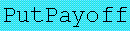`

With an American put option it is quite possible that the value of the underlying stock can drop low enough that the natural upward drift of the stock price will make it profitable to exercise the option early. Indeed, a significant component of the put option's value can be associated with the potential for early exercise. The simplest way to model the option that enables one to consider the possibility of early exercise explicitly is the binominal model. The binomial model divides the time until expiration into a number of equal time periods and over each time segment considers two possibilities, that the stock move either up in price by a fixed proportion or down in price by a fixed proportion. The size of the up and down movements as well as their probability can be chosen so that in the limit as the number of periods approaches infinity, the distribution of prices will converge to the lognormal distribution used in the Black-Scholes model. The derivation of these up and down movements and their probabilities is given in Hull (1989) and are used here within further discussion.

As with the Black-Scholes model, the value of the option is known at expiration and can be determined recursively by working backwards until the present is reached. The difference is that at each point in time the potential advantage of exercising the option immediately must be considered.

The approach that we will take to computing the binomial model is to embed it in a more general framework that can handle far more complex options. This framework casts the process by which stock prices change and the option holder considers his or her alternatives at each point in time as a financial decision tree. Although every option valuation method may generate a different financial decision tree, every tree can be evaluated using the same TreeValue function. Hence, the process for valuing an option will be to convert it into a tree and then evaluate that tree.

The conversion of an American option into a financial decision tree is performed with the MakeAmerTree function which is defined as follows:

```MakeAmerTree[option_,n_:4] :=
Block[{ChanceSymbol = Unique["cnode"],
DecisionSymbol = Unique["dnode"],
s = AssetPrice[option],
k = ExercisePrice[option],
ex = ExerciseFunction[option],
t = ExpirationTime[option],
sd= AssetVolatility[option] Sqrt[t/n] // N,
a = Exp[RiskFreeRate t/n] // N,
u = Exp[sd],
d = 1/u},
ConsObj[Evaluate[ChanceSymbol],
{Type,"chance",
Dfactor,1/a,
Upamt,u,
Downamt,d,
Upprob,(a-d)/(u-d) // N,
ExercisePrice,k,
ExerciseFunction,ex,
Succsym,DecisionSymbol}];
ConsObj[Evaluate[DecisionSymbol],
{Type,"decision",
ExercisePrice,k,
ExerciseFunction,ex,
Succsym,ChanceSymbol}];
node[ChanceSymbol,s,n,1]]
```

This rather imposing function is essentially a list of ingredients needed to build a financial decision tree for an American option. It starts by using the built-in Mathematica function Unique to create symbols for the two types of nodes in the tree, chance nodes, which reflect the up and down movement in the stock price, and decision nodes, which reflect the ability of the optionholder to choose whether or not to exercise the option. The ConsObj function is then used to assign the properties needed by chance and decision nodes to their symbols, ChanceSymbol and DecisionSymbol, respectively. Finally, it creates the seed of the tree as an expression with node as its head and the beginning state of the financial decision tree, including the stock price and period remaining as its body. For expository purposes, MakeAmerTree defaults to four periods, which is enough to understand what it is doing, but not enough for an accurate valuation, which can require ten or more periods.

Let us dive into things by applying MakeAmerTree to DECQL as follows:

`DECQLTree = MakeAmerTree[DECQL]`
`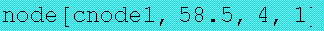`

Hence, we start the tree at a chance node called cnode1 at a stock price of 58.5 with 4 periods to go and a "weight" of 1. (The weight only provides useful information at decision nodes, as we shall see.) To find out where we can proceed from this root node, we need a Successors function that derives a list of successors to both chance and decision nodes. Here is the definition of that function for each type of node:

```Successors[node[symbol_,price_,left_,weight_]] :=
{node[Succsym[symbol],
Upamt[symbol]*price,left-1,
Upprob[symbol]],
node[Succsym[symbol],
Downamt[symbol]*price,left-1,
1-Upprob[symbol]]}  /;   Type[symbol]=="chance" ;

Successors[node[symbol_,price_,left_,weight_]] :=
{node[Succsym[symbol],
price,
left,
1],
node[Succsym[symbol],
price,
0,
1]}                 /; Type[symbol]=="decision"```
` `

We can then simply apply the Successors function to the root node, DECQLTree and see what happens:

`Successors[DECQLTree]`
```     {node[dnode1, 63.3355, 3, 0.499051],
node[dnode1, 54.0336, 3, 0.500949]}```

The successors of this chance node are two decision nodes, both with the symbol dnode1, but reflecting different states of nature. The first decision node corresponds to a DEC stock price of 63.3355, which will occur with a probability/weight of 0.499051. The second decison node corresponds to a DEC stock price of 54.0336 which will occur with a probability/weight of 0.5000949. The "rolling of the dice" associated with the chance node also consumed one period, leaving three to go.

As a quick exercise we can also look directly at the successors of the first decision node as follows:

`Successors[First[Successors[DECQLTree]]]`
`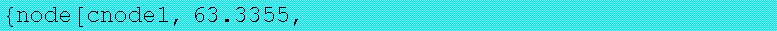`

At a decison node, we are given a choice of continuing to hold the option, leaving periods left set to three, or exercising the option by setting the periods left to zero.

The function TreeValue automates the process of generating successors and simultaneously computes the mathematical expectation for each chance node and chooses the maximum expected payoff for each decision node. This function and its two auxiliary functions, Prob and Expectation, are defined as follows:

```TreeValue[node[symbol_,price_,left_,weight_]] :=
(TreeValue[node[symbol,price,left,weight]]
= Which[left==0,
ExerciseFunction[symbol] [price,ExercisePrice[symbol]],
Type[symbol]=="chance",
Dfactor[symbol] *
Expectation[Successors[node[symbol,price,left,weight]]],
Type[symbol]=="decision",
Max[TreeValue[Successors[node[symbol,price,left,weight]]]]]);

SetAttributes[TreeValue,Listable]

Prob[node_] := node[] ; SetAttributes[Prob,Listable]

Expectation[nodelist_] := Dot[Prob[nodelist],
TreeValue[nodelist]]  ```

The TreeValue function uses Which to distinguish three situations, terminal nodes (left==0), chance nodes, and decision nodes. TreeValue is designed to remember old values so that dynamic programming is employed to reduce the number of evaluations that are required. The downside of this approach is that without additional memory management, memory will tend to fill with the results of old option valuations.

Now that TreeValue has been defined we can apply it to DECQLTree as follows:

`TreeValue[DECQLTree]`
`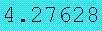`

We can get a more accurate value by expanding the size of the tree that is generated as follows:

`TreeValue[MakeAmerTree[DECQL,8]]`
`     4.27374`

Finally, we can return to the Value function introduced at the end of the previous section and extend its definition to handle all American options on stock that do not pay dividends as follows:

```Value[option_] := BlackScholes[AssetPrice[option],
ExercisePrice[option],
AssetVolatility[option],
RiskFreeRate,
ExpirationTime[option]] /;
Type[option]=="call"

Value[option_] := TreeValue[MakeAmerTree[option,8]] /;
Type[option]=="put"```

This function will now simply apply the Black-Scholes formula to call options and will grow and evaluate a binomial tree for put options. Hence, we can apply it to the two DEC options as follows:

`Value[DECFL]`
``

`Value[DECQL]`
`     4.27374`
` `

### Concluding Remarks

This chapter has provided an extensive introduction to closed-form and numerical approaches to option valuation using Mathematica. Because of its versatile nature, Mathematica is well-suited to the large variety of techniques that may be needed to value options and securities with option components to them. The motivated reader will find it easy to use Mathematica to extend the basic methods developed here to the wide range of option valuation techniques that have been developed in the financial literature. Elsewhere (Miller, 1991) the author has addressed the problem of additional ways of using rule-based methods to extend and build a more "intelligent" object-oriented valuation function.

### References

Black, Fisher, and Myron Scholes. 1973. "The Pricing of Options and Corporate Liabilities," Journal of Political Economy, 81, 637-659.

Cox, John C. and Mark Rubinstein. 1985. Options Markets: Englewood Cliffs (NJ), Prentice-Hall.

Hull, John. 1989. Options, Futures and Other Derivative Securities: Englewood Cliffs (NJ), Prentice-Hall.

Miller, Ross M. 1990b. "Computer-Aided Financial Analysis: An Implementation of the Black-Scholes Model," Mathematica Journal, 1, 75-79.

Miller, Ross M. 1991. "An Intelligent Approach to Financial Valuation," Proceedings of the First International Conference on Artificial Intelligence Applications on Wall Street, IEEE Press.

### Author Biography

Ross M. Miller received his B.S. in mathematics from the California Institute of Technology and his A.M. and Ph.D. in economics from Harvard University. He served on the faculties of Boston University, the California Institute of Technology, and the University of Houston before taking his present position as a senior member of the technical staff of the GE Corporate Research & Development Center. Dr. Miller is author of the book Computer-Aided Financial Analysis and has published extensively on information transfer in financial markets and on the design of advanced electronic market systems.